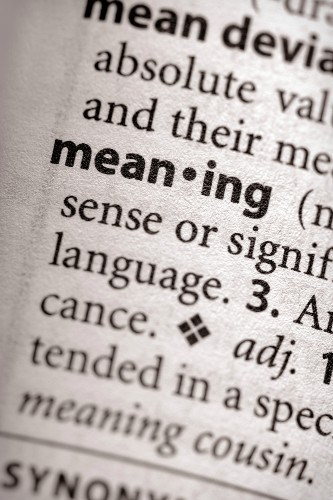## About The Word Wave equationLearn about the word Wave equation to help solve your crossword puzzle. Discover Wave equation definitions and meaning, origins, synonyms, related terms and more at the free Crossword Dictionary.

## Wave equationWave equation Definition And Meaning

### What's The Definition Of Wave equation?

[n] a differential equation that describes the passage of harmonic waves through a medium

Synonyms | Synonyms for Wave equation:

Related Terms | Find terms related to Wave equation: